26 Nov 2013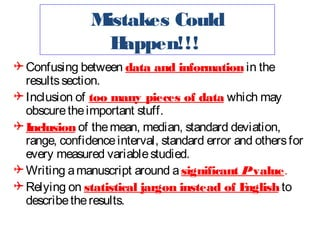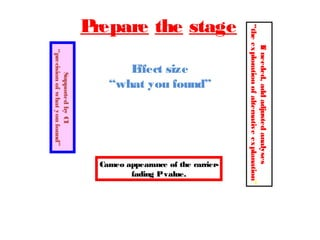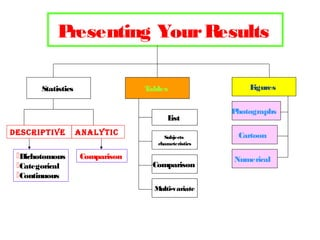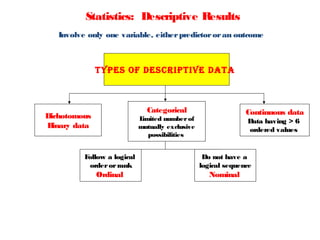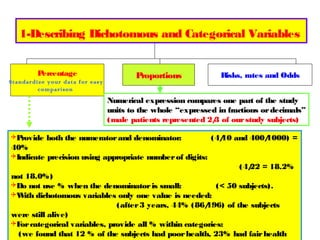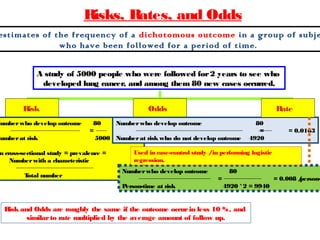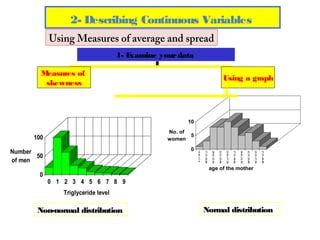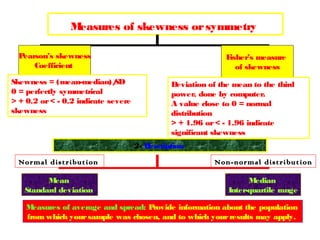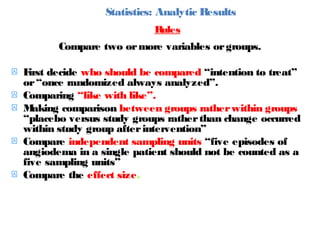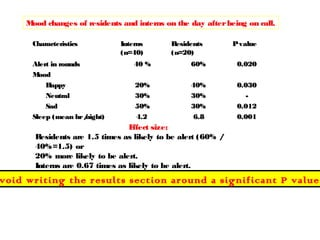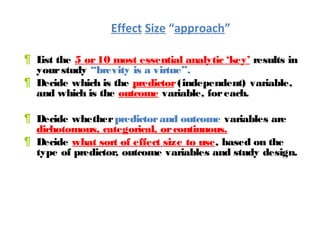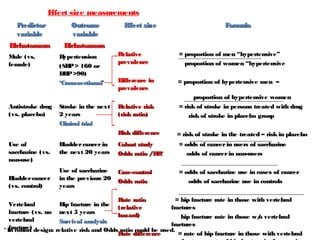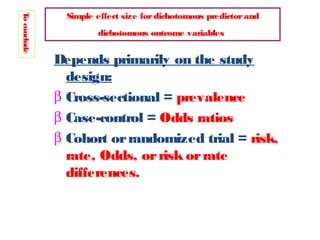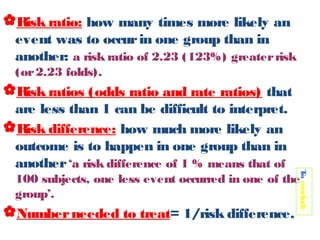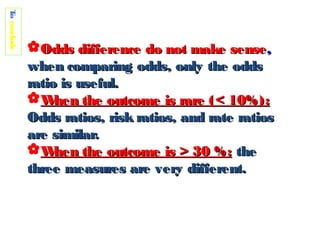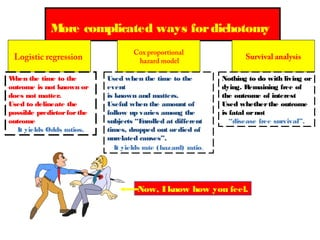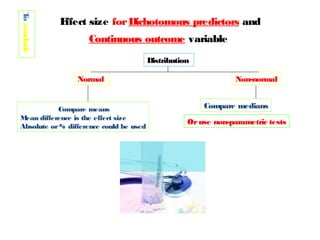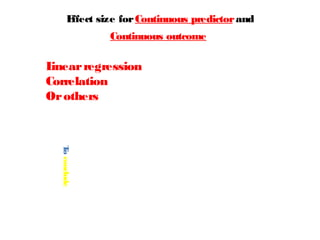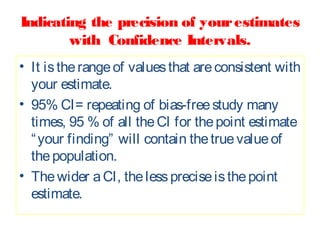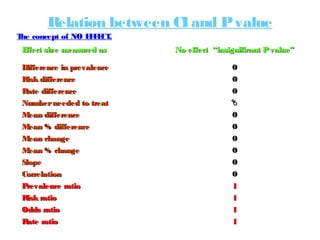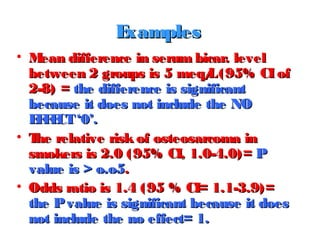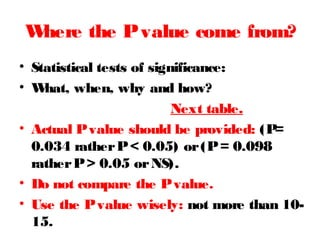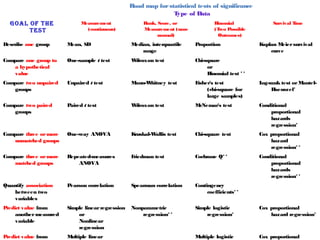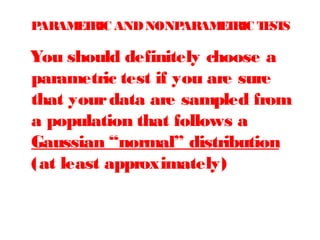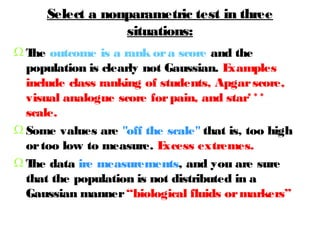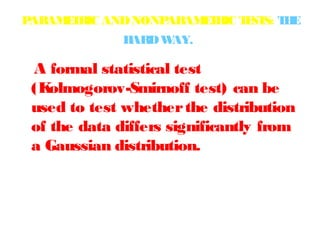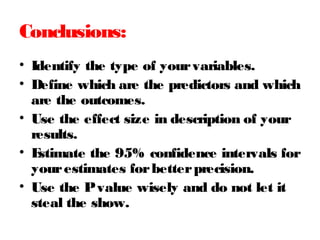1 sur 32

• 1. Displaying Your Results Dr Tarek Amin Professor of Public Health Cairo University amin55@myway.com
• 2. Are you Currently engaged in research? Intention to publish in indexed journals?
• 3. M istakes Could H appen!!!  Confusing between data and information in the results section.  Inclusion of too many pieces of data which may obscure the important stuff.  Inclusion of the mean, median, standard deviation, range, confidence interval, standard error and others for every measured variable studied.  Writing a manuscript around a significant P value.  Relying on statistical jargon instead of E nglish to describe the results.
• 4. Supported by CI “precision of what you found” E ffect size “what you found” Cameo appearance of the carrierfading P value. If needed, add adjusted analyses “the exploration of alternative explanation” P repare the stage
• 5. P resenting Your Results Statistics T ables L ist Descriptive Dichotomous Categorical Continuous AnAlytic Comparison Subjects characteristics Comparison M ulti-variate F igures P hotographs Cartoon Numerical
• 6. Statistics: Descriptive Results Involve only one variable, either predictor or an outcome types of Descriptive DAtA Categorical Dichotomous B inary data Continuous data L imited number of mutually exclusive possibilities Data having > 6 ordered values F ollow a logical order or rank Do not have a logical sequence Ordinal Nominal
• 7. 1-Describing Dichotomous and Categorical Variables P ercentage Standardize your data for easy comparison P roportions Risks, rates and Odds Numerical expression compares one part of the study units to the whole “expressed in fractions or decimals” (male patients represented 2/ of our study subjects) 3 P rovide both the numerator and denominator: (4/ and 400/ 10 1000) = 40% Indicate precision using appropriate number of digits: (4/ = 18.2% 22 not 18.0%) Do not use % when the denominator is small: (< 50 subjects). W dichotomous variables only one value is needed: ith (after 3 years, 44% (86/ 196) of the subjects were still alive) F categorical variables, provide all % within categories: or (we found that 12 % of the subjects had poor health, 23% had fair health
• 8. Risks, Rates, and Odds estimates of the frequency of a dichotomous outcome in a group of subje who have been followed for a period of time. A study of 5000 people who were followed for 2 years to see who developed lung cancer, and among them 80 new cases occurred. Risk Number who develop outcome Number at risk Odds 80 Number who develop outcome = = 0.016 5000 Number at risk who do not develop outcome In cross-sectional study = prevalence = Number with a characteristic T otal number R ate 80 = 4920 = 0.0163 Used in case-control study /in performing logistic regression. Number who develop outcome 80 = P erson-time at risk = 0.008 / person4920 * 2 = 9940 Risk and Odds are roughly the same if the outcome occur in less 10 %, and similar to rate multiplied by the average amount of follow up.
• 9. Going little more deeper
• 10. 2- Describing Continuous Variables Using Measures of average and spread 1- E xamine your data M easures of skewness Using a graph 10 No. of 5 women 100 37.51-40.00 35.51-37.50 32.51-35.00 30.01-32.50 27.51-30.00 25.01-27.50 22.51-25.00 20.01-22.50 17.51-20.00 0 15.01-17.5 0 Number 50 of men age of the mother 0 1 2 3 4 5 6 7 8 9 Triglyceride level Non-normal distribution Normal distribution
• 11. M easures of skewness or symmetry Pearson’s skewness Coefficient Skewness = (mean-median)/ SD 0 = perfectly symmetrical > + 0.2 or < - 0.2 indicate severe skewness F isher’s measure of skewness Deviation of the mean to the third power, done by computer. A value close to 0 = normal distribution > + 1.96 or < - 1.96 indicate significant skewness 2- Description Normal distribution Non-normal distribution M ean Standard deviation M edian Inter-quartile range M easures of average and spread: P rovide information about the population from which your sample was chosen, and to which your results may apply.
• 12. Statistics: Analytic Results Rules Compare two or more variables or groups.  F decide who should be compared “intention to treat” irst or “once randomized always analyzed”.  Comparing “like with like”.  M aking comparison between groups rather within groups “placebo versus study groups rather than change occurred within study group after intervention”  Compare independent sampling units “five episodes of angiodema in a single patient should not be counted as a five sampling units”  Compare the effect size.
• 13. M ood changes of residents and interns on the day after being on call. Characteristics Alert in rounds M ood H appy Neutral Sad Sleep (mean hr / night) Interns (n=40) Residents (n=20) P value 40 % 60% 0.020 20% 30% 50% 4.2 40% 30% 30% 6.8 0.030 0.012 0.001 E ffect size: Residents are 1.5 times as likely to be alert (60% / 40%=1.5) or 20% more likely to be alert. Interns are 0.67 times as likely to be alert. void writing the results section around a significant P value.
• 14. Effect Size “approach” ¶ L the 5 or 10 most essential analytic ‘key’ results in ist your study “brevity is a virtue”. ¶ Decide which is the predictor (independent) variable, and which is the outcome variable, for each. ¶ Decide whether predictor and outcome variables are dichotomous, categorical, or continuous. ¶ Decide what sort of effect size to use, based on the type of predictor, outcome variables and study design.
• 15. E ffect size measurements P redictor variable Outcome variable Dichotomous Dichotomous M (vs. ale female) Antistroke drug (vs. placebo) H ypertension (SB > 160 or P DB >90) P ‘Cross-sectional’ Stroke in the next 2 years Clinical trial E ffect size Relative prevalence = proportion of men “hypertensive” proportion of women “hypertensive Difference in prevalence = proportion of hypertensive men – Relative risk (risk ratio) Risk difference Use of saccharine (vs. non-use) B ladder cancer (vs. control) Vertebral fracture (vs. no vertebral fracture) * In cohort design: F ormula proportion of hypertensive women = risk of stroke in persons treated with drug risk of stroke in placebo group = risk of stroke in the treated – risk in placebo B ladder cancer in the next 20 years Cohort study Odds ratio /RR* = odds of cancer in users of saccharine odds of cancer in non-users Use of saccharine in the previous 20 years Case-control Odds ratio = odds of saccharine use in cases of cancer odds of saccharine use in controls Rate ratio = hip fracture rate in those with vertebral H fracture in the (relative ip fractures next 5 years hazard) hip fracture rate in those w/ vertebral o Survival analysis fractures relative risk and Odds ratio could be used. Rate difference = rate of hip fracture in those with vertebral
• 16. E ffect size measurements P redictor variable Outcome variable Dichotomous Continuous W hite (vs. non white) Systolic blood pressure (SB ) P Case-control / Cohort New drug (vs. placebo) Change in diastolic blood pressure (DB ) P Clinical trial Continuous E ffect size M ean difference M ean % difference” F ormula = mean SB in whites – mean SB in non P P whites = mean SB in white – mean SB in non P P whites mean SB in non white P Continuous M ean difference in change Creatinine clearance 1, 25- vitamin D level Regression/ Slope Serum lead level IQ P roportion of variance explained = mean change in DB in treatment group – P mean change in DB in placebo group P Change in 1, 25 Vitamin D level per change in creatinine clearance Correlation between lead level and IQ
• 17. T conclude o Simple effect size for dichotomous predictor and dichotomous outcome variables Depends primarily on the study design: β Cross-sectional = prevalence β Case-control = Odds ratios β Cohort or randomized trial = risk, rate, Odds, or risk or rate differences.
• 18. Risk ratio: how many times more likely an event was to occur in one group than in another: a risk ratio of 2.23 (123%) greater risk (or 2.23 folds). T conclude o Risk ratios (odds ratio and rate ratios) that are less than 1 can be difficult to interpret. Risk difference: how much more likely an outcome is to happen in one group than in another ‘a risk difference of 1 % means that of 100 subjects, one less event occurred in one of the group’. Number needed to treat= 1/risk difference.
• 19. T conclude o Odds difference do not make sense , when comparing odds, only the odds ratio is useful. W hen the outcome is rare (< 10%): Odds ratios, risk ratios, and rate ratios are similar. W hen the outcome is > 30 %: the three measures are very different.
• 20. M ore complicated ways for dichotomy Logistic regression W hen the time to the outcome is not known or does not matter. Used to delineate the possible predictor for the outcome It yields Odds ratios. Cox proportional hazard model Used when the time to the event is known and matters. Useful when the amount of follow up varies among the subjects “E nrolled at different times, dropped out or died of unrelated causes”. It yields rate (hazard) ratio. Survival analysis Nothing to do with living or dying. Remaining free of the outcome of interest Used whether the outcome is fatal or not “disease free survival”. Now, I know how you feel.
• 21. T conclude o E ffect size for Dichotomous predictors and Continuous outcome variable Distribution Normal Compare means M ean difference is the effect size Absolute or % difference could be used Non-normal Compare medians Or use non-parametric tests
• 22. E ffect size for Continuous predictor and Continuous outcome L inear regression Correlation Or others T conclude o
• 23. Indicating the precision of your estimates with Confidence Intervals. • It is the range of values that are consistent with your estimate. • 95% CI= repeating of bias-free study many times, 95 % of all the CI for the point estimate “ your finding” will contain the true value of the population. • The wider a CI, the less precise is the point estimate.
• 24. Relation between CI and P value T concept of NO E F CT he FE . E ffect size measured as No effect “insignificant P value” Difference in prevalence Risk difference Rate difference Number needed to treat M ean difference M ean % difference M ean change M ean % change Slope Correlation P revalence ratio Risk ratio Odds ratio Rate ratio 0 0 0 ზ 0 0 0 0 0 0 1 1 1 1
• 25. E xamples • M ean difference in serum bicar. level between 2 groups is 5 meq/ (95% CI of L 2-8) = the difference is significant because it does not include the NO E F CT ‘0’. FE • T relative risk of osteosarcoma in he smokers is 2.0 (95% CI, 1.0-4.0)= P value is > o.o5. • Odds ratio is 1.4 (95 % CI= 1.1-3.9)= the P value is significant because it does not include the no effect= 1.
• 26. W here the P value come from? • Statistical tests of significance: • W hat, when, why and how? Next table. • Actual P value should be provided: (P = 0.034 rather P < 0.05) or (P = 0.098 rather P > 0.05 or NS). • Do not compare the P value. • Use the P value wisely: not more than 1015.
• 27. Road map for statistical tests of significance T ype of Data Goal of the test Measurement (continuous) Rank, Score, or M easurement (nonnormal) B inomial (T P wo ossible Outcomes) Survival T ime Describe one group M ean, SD M edian, interquartile range P roportion K aplan M eier survival curve Compare one group to a hypothetical value One-sample t test W ilcoxon test Chi-square or B inomial test * * Compare two unpaired groups Unpaired t test M ann-W hitney test F isher's test (chi-square for large samples) L og-rank test or M antelH aenszel* Compare two paired groups P aired t test W ilcoxon test M cNemar's test Conditional proportional hazards regression* Compare three or more unmatched groups One-way ANOVA K ruskal-W allis test Chi-square test Cox proportional hazard regression* * Compare three or more matched groups Repeated-measures ANOVA F riedman test Cochrane Q* * Conditional proportional hazards regression* * Quantify association between two variables P earson correlation Spearman correlation Contingency coefficients* * P redict value from another measured variable Simple linear regression or Nonlinear regression Nonparametric regression* * Simple logistic regression* Cox proportional hazard regression* P redict value from M ultiple linear M ultiple logistic Cox proportional
• 28. PARAM T E RIC AND NONP ARAM T E RIC T ST E S You should definitely choose a parametric test if you are sure that your data are sampled from a population that follows a Gaussian “normal” distribution (at least approximately)
• 29. Select a nonparametric test in three situations: Ω T outcome is a rank or a score and the he population is clearly not Gaussian. E xamples include class ranking of students, Apgar score, visual analogue score for pain, and star* * * scale. Ω Some values are "off the scale" that is, too high or too low to measure. E xcess extremes. Ω T data ire measurements, and you are sure he that the population is not distributed in a Gaussian manner “biological fluids or markers”
• 30. P ARAM T E RIC AND NONP ARAM T E RIC T ST T E E S: H H ARD W AY. A formal statistical test (K olmogorov-Smirnoff test) can be used to test whether the distribution of the data differs significantly from a Gaussian distribution.
• 31. Conclusions: • Identify the type of your variables. • Define which are the predictors and which are the outcomes. • Use the effect size in description of your results. • E stimate the 95% confidence intervals for your estimates for better precision. • Use the P value wisely and do not let it steal the show.
• 32. Thank you